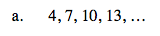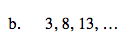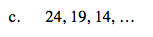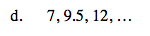### Home > A2C > Chapter 2 > Lesson 2.1.5 > Problem2-73

2-73.
1. Find the common difference for each sequence listed below. Write an expression for the nth term in each sequence below, keeping in mind that the first term of each sequence is t(0). Homework Help ✎

1. 4, 7, 10, 13, …

2. 3, 8, 13, …

3. 24, 19, 14, …

4. 7, 9.5, 12, …7 − 4 = 10 − 7 = 13 − 10 = 3

m = 3

t(n) = 3n + t(0)

t(0) = 4

t(n) = 3n + 4m = 5

t(n) = 5n + 3m = −5

t(n) = −5n + 249.5 − 7 = 12 − 9.5 = 2.5

m = 2.5

t(0) = 7

t(n) = 2.5n + 7